# Hysteron

(diff) ← Older revision | Latest revision (diff) | Newer revision → (diff)

Only a very simple modification of the non-linearity "hysteron" is described below. See [a1] for the general definition and an identification theorem, that is, qualitative conditions under which a "black box" is a hysteron. Consider in the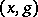-plane the graphs of two continuous functions,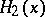satisfying the inequality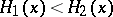,. Suppose that the set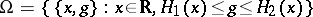is partitioned into the disjoint union of the continuous family of graphs of continuous functions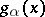, whereis a parameter. Each functionis defined on its interval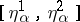,, and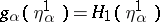,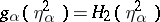, that is, the end-points of the graphs of the functionsbelong to the union of the graphs ofand(see Fig.a2.).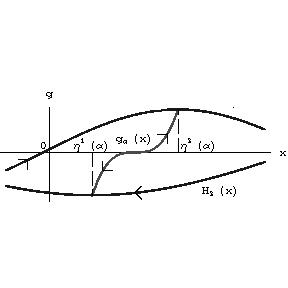Figure: h110430a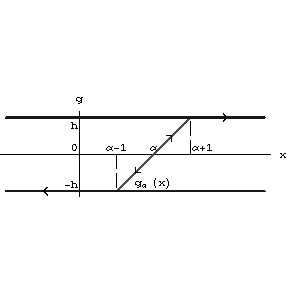Figure: h110430b

Hysteron: Prandtl non-linearity

A hysteron is a transducer with internal statesfrom the segmentand with the following input–output operators. The variable output() is defined by the formula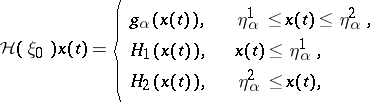for monotone inputs,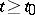. The value ofis determined by the initial stateto satisfyand the corresponding variable internal state is defined by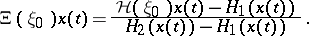For piecewise-monotone continuous inputs the output is constructed by the semi-group identity. The input–output operators can then be extended to the totality of all continuous inputs by continuity (see [a1]). The operators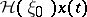,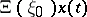are defined for each continuous input and each initial state. They are continuous as operators in the space of continuous functions with the uniform metric (cf. also Metric).

A hysteron is called a Prandtl non-linearity if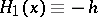,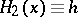;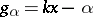,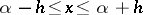,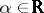. This non-linearity describes the Prandtl model of ideal plasticity with Young modulusand elastic limits. The parallel connections of a finite numbers of such elements describe the Besseling model of elasto-plasticity and the continual counterpart describe the Ishlinskii model.